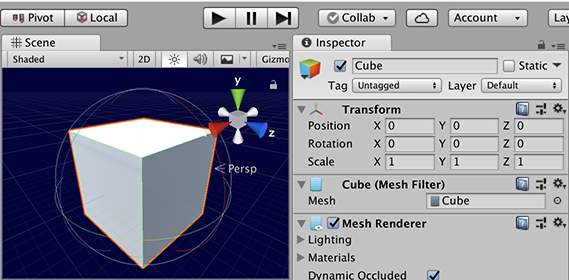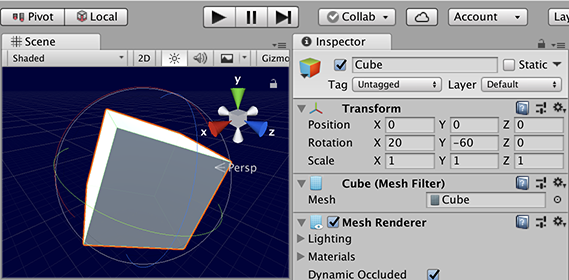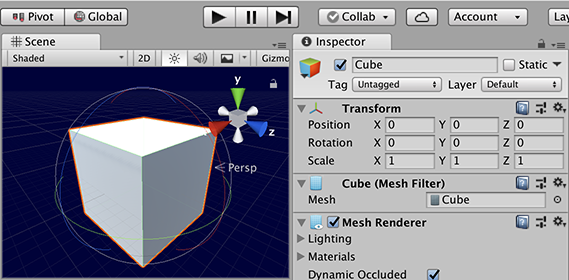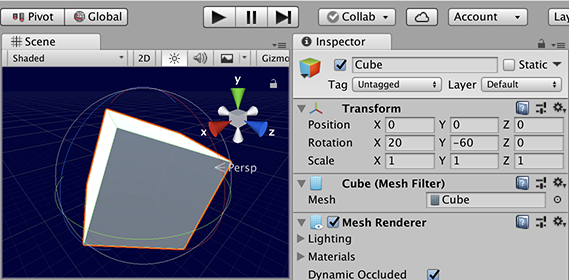LanguageEnglish
• C#

Transform.Rotate

Suggest a change

Success!

Thank you for helping us improve the quality of Unity Documentation. Although we cannot accept all submissions, we do read each suggested change from our users and will make updates where applicable.

Close

Submission failed

For some reason your suggested change could not be submitted. Please <a>try again</a> in a few minutes. And thank you for taking the time to help us improve the quality of Unity Documentation.

Close

Cancel

Switch to Manual

Description

Use Transform.Rotate to rotate GameObjects in a variety of ways. The rotation is often provided as a Euler angle and not a Quaternion.

You can specify a rotation in world axes or local axes.

World axis rotation uses the coordinate system of the Scene, so when you start rotate a GameObject, its x, y, and z axes are aligned with the x, y, and z world axes. So if you randomly rotate a cube in world space, its axes align with the world. When you select a cube in the Unity Editor’s Scene view, rotation Gizmos appear for the left/right, up/down and forward/back rotation axes. Moving these Gizmos rotates the cube around the axes. If you de-select and then re-select the cube, the axes restart in world alignment.

Local rotation uses the coordinate system of the GameObject itself. So, a newly created cube uses its x, y, and z axis set to zero rotation. Rotating the cube updates the rotation axes. If you de-select and the re-select the cube, the axes will be shown in the orientation they were before.A cube not rotated in Local Gizmo ToggleA cube rotated in Local Gizmo ToggleA cube not rotated in Global Gizmo ToggleA cube rotated in Global Gizmo Toggle

public void Rotate(Vector3 eulers, Space relativeTo = Space.Self);

Parameters

 eulers The rotation to apply. relativeTo Determines whether to rotate the GameObject either locally to the GameObject or relative to the Scene in world space.

Description

Applies a rotation of eulerAngles.z degrees around the z-axis, eulerAngles.x degrees around the x-axis, and eulerAngles.y degrees around the y-axis (in that order).

Rotate takes a Vector3 argument as a euler angle. The second argument is the rotation axes, which can be set to local axis (Space.Self) or global axis (Space.World). The rotation is by the Euler amount.

public void Rotate(float xAngle, float yAngle, float zAngle, Space relativeTo = Space.Self);

Parameters

 relativeTo Determines whether to rotate the GameObject either locally to the GameObject or relative to the Scene in world space. xAngle Degrees to rotate the GameObject around the X axis. yAngle Degrees to rotate the GameObject around the Y axis. zAngle Degrees to rotate the GameObject around the Z axis.

Description

Applies a rotation of zAngle degrees around the z axis, xAngle degrees around the x axis, and yAngle degrees around the y axis (in that order).

Rotate can have the euler angle specified in 3 floats for x, y, and z.

The example shows two cubes: one cube uses Space.Self (the local space and axes of the GameObject) and the other uses Space.World (the space and axes in relation to the Scene). Both cubes have the same angle using 3 Scenes. When two or three of the Scenes are given values of approximately 0.25f, both cubes will rotate. The X ValueSceneZ Value Inspector fields store the rotation values. The cubes rotate in different ways. To see the difference, add the script to your Scene and take a look at the Scene view as the Game is running. In the Scene view, make sure that Local is set by the Gizmo Toggle, and select the Self cube. The Self cube rotates with the x, y and z arrows continuing their direction through the cube faces. Now change the Gizmo Toggle to Global and select the World cube. The is aligned with the world x, y and z axes.

using UnityEngine;

// Transform.Rotate example // // Two cubes are created. One (red) is rendered using Space.Self. The // other (green) uses Space.World. The rotation is controlled using xAngle, // yAngle and zAngle. Over time, the cubes rotate differently.

public class ExampleScript : MonoBehaviour { public float xAngle, yAngle, zAngle; public Material selfMat, worldMat;

private GameObject cube1, cube2;

void Awake() { cube1 = GameObject.CreatePrimitive(PrimitiveType.Cube); cube1.transform.position = new Vector3(0.75f, 0.0f, 0.0f); cube1.GetComponent<Renderer>().material = selfMat; cube1.name = "Self";

cube2 = GameObject.CreatePrimitive(PrimitiveType.Cube); cube2.transform.position = new Vector3(-0.75f, 0.0f, 0.0f); cube2.GetComponent<Renderer>().material = worldMat; cube2.name = "World"; }

void Update() { cube1.transform.Rotate(xAngle, yAngle, zAngle, Space.Self); cube2.transform.Rotate(xAngle, yAngle, zAngle, Space.World); } }

public void Rotate(Vector3 axis, float angle, Space relativeTo = Space.Self);

Parameters

 angle The degrees of rotation to apply. axis The axis to apply rotation to. relativeTo Determines whether to rotate the GameObject either locally to the GameObject or relative to the Scene in world space.

Description

Rotates the object around the given axis by the number of degrees defined by the given angle.

Rotate has an axis, angle and the local or global parameters. The rotation axis can be in any direction.